# Notes on BRST VI: Casimir Operators

For the case of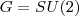, it is well-known from the discussion of angular momentum in any quantum mechanics textbook that irreducible representations can be labeled either by j, the highest weight (here, highest eigenvalue of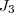), or by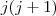, the eigenvalue of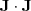. The first of these requires making a choice (the z-axis) and looking at a specific vector in the representation, the second doesn’t. It was a physicist (Hendrik Casimir), who first recognized the existence of an analog offor general semi-simple Lie algebras, and the important role that this plays in representation theory.

The Casimir Operator

Recall that for a semi-simple Lie algebra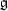one has a non-degenerate, invariant, symmetric bi-linear form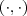, the Killing form, given by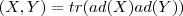If one starts withthe Lie algebra of a compact group, this bilinear form is defined on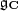, and negative-definite on. For a simple Lie algebra, taking the trace in a different representation gives the same bilinear form up to a constant. As an example, for the case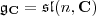, one can show that

(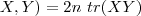here taking the trace in the fundamental representation asbycomplex matrices.
One can use the Killing form to define a distinguished quadratic element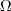of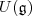, the Casimir element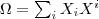where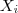is an orthonormal basis with respect to the Killing form and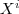is the dual basis. On any representation, this gives a Casimir operator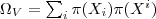Note that, taking the representationto be the space of functions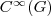on the compact Lie group G,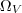is an invariant second-order differential operator, (minus) the Laplacian.is independent of the choice of basis, and belongs to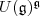, the subalgebra ofinvariant under the adjoint action. It turns out that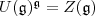, the center of. By Schur’s lemma, anything in the center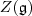must act on an irreducible representation by a scalar. One can compute the scalar for an irreducible representation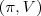as follows:

Choose a basis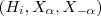ofwith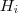an orthonormal basis of the Cartan subalgebra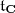, and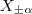elements of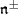in the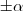root-spaces of, orthonormal in the sense of satisfying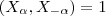Then one has the following expression for: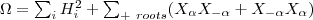To compute the scalar eigenvalue of this on an irreducible representation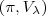of highest weight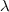, one can just act on a highest weight vector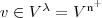. On this vector the raising operators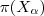act trivially, and using the commutation relation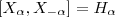(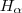is the element of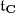satisfying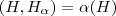) one finds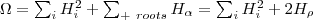where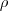is half the sum of the positive roots, a quantity which keeps appearing in this story. Acting on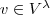one finds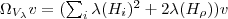Using the inner-product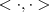induced on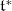by the Killing form, this eigenvalue can be written as: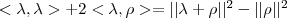In the special case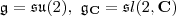, there is just one positive root, and one can take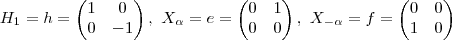Computing the Killing form, one findsand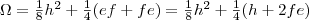On a highest weight vectoracts as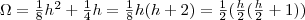This is 1/2 times the physicist’s operator, and in the irreducible representation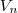of spin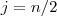, it acts with eigenvalue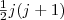.

In the next posting in this series I’ll discuss the Harish-Chandra homomorphism, and the question of how the Casimir acts not just on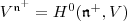, but on all of the cohomology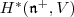. After that, taking note that the Casimir is in some sense a Laplacian, we’ll follow Dirac and introduce Clifford algebras and spinors in order to take its square root.

This entry was posted in BRST. Bookmark the permalink.

### 8 Responses to Notes on BRST VI: Casimir Operators

1. Tony Smith says:

Peter, will you be discussing the physical significance of all the Casimir operators of all groups used in physics model-building,
or will your discussion be restricted to the quadratic Casimir ?

Tony Smith

2. woit says:

Tony,

The next topic, the Harish-Chandra homomorphism, deals with the story of the higher-order Casimirs. But after that, what I’m writing about deals with Dirac operators. In some sense, from then on, the story is about the square root of the quadratic Casimir, not the higher order ones.

3. Coin says:

Woit: Small note, looking at the listing for your BRST category I find that BRST IV got left out, you may want to fix that.

4. James says:

I’m sorely missing my usual fix of “scandal-mongering or stirring up trouble of one kind or another”, and after some months of cold-turkey and BRST treatment, I wonder if you could explain the motivation, and long-term prognosis, of all of this group-therapy?

As an outsider I would ask: is this some technique for creating new potential QM theories, or a way of quantising classical models, or to try to put existing QM theories on a surer maths footing, or is it just cute maths in itself?

5. Peter Woit says:

Coin,

Fixed.

James,

I’m back from a trip, and have tried to do a bit more stirring up of trouble. Unfortunately, it’s getting harder to stir up trouble in this area. Nothing much new is happening, and I and pretty much everyone else is tired of the same old topics.

Now that I’m back, and classes are over for the semester, I’ll try to pick up the pace, and get to the point with the BRST postings, ending with some more explicit speculation about what all this might be useful for. Still in the realm of speculation, it might be useful for getting some sort of new insight into the non-perturbative quantization of gauge theories such as the standard model, as well as useful in purely mathematical problems about representation theory.

6. D R Lunsford says:

Peter, am I right that SO(3,3) has Casimirs of rank 2,3, and 4? Looking forward to the next installment.

-drl

7. woit says:

drl,

The Lie algebra of SO(3,3) is a real form of the Lie algebra of SO(6,C)=SL(4,C). Just finished writing up the next posting, where I at least mention that the higher Casimirs of sl(n,C) are of degree, 2, 3, etc. up to n, so, yes in your case they are of degree 2,3 and 4.